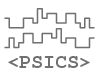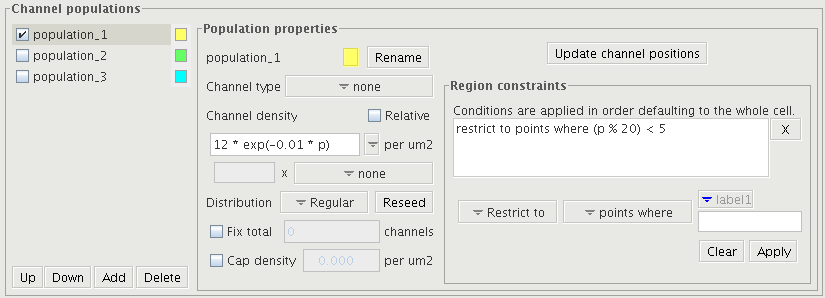PSICS - the Parallel Stochastic Ion Channel Simulator
previous ←   → next
Under development
Please let us know of errors, unclear text or if you have suggestions form improvements:# Specifying channel distributions

The ICING user interface provides an alternative method for specifying channel distributions instead of writing the xml by hand. The two methods allow the same set of models to be expressed but ICING does not directly match the layout of the ChannelPopulation block and its children. Instead, it intended to be accessible with minimal reference to the formats documentation. Specifications created with ICING are, however, stored in exactly the same format as those created by hand. Hand-edited models can be opened in ICING and vice versa without any difficulty.The image shows the channel distribution panel. The left column is for selecting the population to edit. The checkboxes to the left set whether it should be shown in the morphology display. The center specifies how the local density is calculated and the right column sets the region on the cell over which the density applies.

## Density specification

The simplest way to set the density is with a constant value in the Chanel density field (in channels per square micron) and all the checkboxes unselected. For variable densities, this field can contain an expression in terms of the path length, p, local radius r, diameter, d and branch order b. The expression uses standard programming style rather than mathematical notation in that "*" signs are required for multiplication (whereas they are normally implicit in mathematical notation) and functions are written out as abbreviated words: exp, log, abs, sin, cos, tan, log10.

Possible density expessinos include
10
10 * exp (-0.01 * p)
5. / (p + 10) + 2
4 * r * r

After specifying the density per unit area, there is still some freedom in how this is interpreted when positioning channels. There are two supported options provided by the Distribution drop-down. If set to Regular then channels are placed at uniform intervals along each process. If set to Poisson then the density is interpreted as a probability of finding a channel in the next small increment along the dendrite. In either case, the angle around the process is sampled from a uniform distribution. The seed for random channel allocations is stored as part of the specification so that the positions used in the calculation are exactly those shown in ICING. The Reseed button resets the seed allowing a new set of positions to be generated.

The density set by the expression can be modified in two ways. The Cap density field sets an upper limit, and the Fix total option rescales the applied density to give the desired total number of channels on the cell. Capping the density can be useful to avoid unwanted behavior of expressions outside the region they are intended for - at the origin for a 1/p rule, for example, or in remote parts of the structure with a rule such as "increase linearly away from the soma up to 50 channels per um2".

### Relative densities

A central aim of the PSICS xml formats is to minimize redundancy or duplication in the specifications. To this end, if several channel populations have the same distribution profile up to a scaling factor, then the distribution rule can be specified once and then each population can make reference to the single instance of the rule. For exploratory modelling this is normally what is required instead of having multiple instances of the parameters that all need changing together. For example, where certain groups of channels act together (like sodium and potassium channels in the HH model) the the behavior is typically much more sensitive to changes in just one of the populations than to scaling then both up or down together.

ICING gives the same effect via relative densities. This uses a canonical channel population to set the density expression and then lets other populations set their densities a a fixed factor times the density of the first population. It is achieved by selecting the Relative checkbox for the channel density and then setting the scaling factor and target channel population.

## Region constraints

The region over which a distribution is applied is taken, by default, to be the whole cell. This can be changed by adding one or more region constraints. These take the form of stylized sentences specifying an operation (include, exclude, or restrict to) and a region. The region is either relative to labelled points on the structure or, in the case of the where option, it is set by a boolean expression evaluated over the cell.

The applicable region is computed by applying the constraints in order. The include operation causes any points matching the current condition to be added to those already selected. The exclude operation removes any matching points from the active set, and the restrict to operation leaves only those points that are both in the active set and match the current condition.

For label-dependent regions, the labels should be positioned on the appropriate structure. The labels available in the user interface are taken from the active morphology. To target specific sections of dendrite two conditions can be used. For example a first condition "include points proximal to label2" with a second one "restrict to points distal to label1" gives just the section between label1 and label2 on the structure.

The quantities available for expressions to use with the "where" condition are the same as for the density expression, except that here the expression must be boolean (true/false) valued rather than real-valued for the density. Examples of such expressions include:

 p < 1000 points less than 100 um from the soma (r > 2) && (p < 20) wide dendrites more than 20um from the soma (p % 100) < 1 1um long sections every 100 microns.

This combination of density expressions, region constraints, capping, labels and fixed totals should enable most distributions of interest to be expressed relatively concisely. If necessary, several population definitions can refer to the same channel type in order to build up a complex density profile as a number of separate pieces.

previous ←   → next